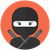# QlikView App Dev

Discussion Board for collaboration related to QlikView App Development.

Announcements
Join this live chat April 6, 10AM EST - QlikView to Qlik Sense REGISTER
cancel
Showing results for
Search instead for
Did you mean:Contributor III

## set analysis / aggr function

Hi gurus

I am having some set analysis / aggr function issues. I am trying to sum some specific values.

Conditions:

• If Area = 34 and total amount within S_ID and Item is under 10
• Then sum amount only for Type AB

There are multiple items and other areas. For the simplicity in the sample, I kept only one.

I want to show Date, Item and the calculation.

As you can see in the sample the total sum of all Type AB’s is 45. However, the 2 lines marked with yellow should not be counted in the calc, since the total amount is above 10 (total and not type specific). The result should be 29 (45-12-4 = 29).Test 3 gives the right result if I add the dimension of S_ID, but not without it.Something in Test 2 do not calculate correct, if both types is present or in case of duplicates (the orange ones).Expression:

Test 1: sum(if(aggr(Sum({<Area={34}>}Amount),S_ID,Item)<10,sum(if(Type='AB',Amount))))

Test 2: sum(if(aggr(Sum({<Area={34}>}Amount),S_ID,Item)<10 and Type='AB',Amount))

Test 3: if(aggr(Sum({<Area={34}>}Amount),S_ID,Item)<10,sum(if(Type='AB',Amount)))

Any ideas of how to accomplice this?

Labels (2)

• ### Set Analysis

1 Solution

Accepted SolutionsMVP

Try this

``Sum(Aggr(If(Sum({<Area = {34}>} Amount) < 10, Sum({<Type = {'AB'}>} Amount)), Date, S_ID, Item))``
2 RepliesMVP

Try this

``Sum(Aggr(If(Sum({<Area = {34}>} Amount) < 10, Sum({<Type = {'AB'}>} Amount)), Date, S_ID, Item))``Contributor III
Author

@sunny_talwar wrote:

Try this

``Sum(Aggr(If(Sum({<Area = {34}>} Amount) < 10, Sum({<Type = {'AB'}>} Amount)), Date, S_ID, Item))``

Nice. This solved the issue. Had to place the Area = {34} in the next sum as well to get the result I needed.

Sum(Aggr(If(Sum({<Area = {34}>} Amount) < 10, Sum({<Area = {34}, Type = {'AB'}>} Amount)), Date, S_ID, Item))

Thank you so much 😀👍